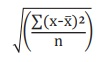Home | | Economics 12th Std | Standard Deviation (σ)

# Standard Deviation (σ)

The measures of central tendency serve to locate the center of the distribution, but they do not reveal how the items are spread out on either side of the center.

Standard Deviation (σ)

The measures of central tendency serve to locate the center of the distribution, but they do not reveal how the items are spread out on either side of the center. This characteristic of a frequency distribution is commonly referred to as dispersion. The degree of variation is evaluated by various measures of dispersion. There are two kinds of measures of dispersion, namely

1. Absolute measure of dispersion

2. Relative measure of dispersion

Absolute measure of dispersion indicates the amount of variation in a set of values in terms of units of observations.

Relative measures of dispersion are free from the units of measurements of the observations. They are pure numbers. They are used to compare the variation in two or more sets, which are having different units of measurements of observations.

Standard Deviation  is  one  of the  methods  of  Absolute  measure  of dispersion. Karl Pearson introduced the concept of standard deviation in 1893. Standard deviation is also called Root- Mean Square Deviation. The reason is that it is the square–root of the mean of the squared deviation from the arithmetic mean. It provides accurate result. Square of standard deviation is called Variance.

## Definition:

It is defined as the positive square-root of the arithmetic mean of the square of the deviations of the given observation from their arithmetic mean.

The standard deviation of the population is denoted by the Greek letter σ (sigma) The standard deviation of sample is denoted as 's'.

## Calculation of Standard deviation-Individual Series:

There are two methods of calculating Standard deviation in an individual series.

a) Deviations taken from Actual mean

b) Deviation taken from Assumed mean

Standard Deviation =Where, x̅ = mean value of distribution

n = number of observations.

Steps:

1. Find  out the actual mean of given data(X̅ )

2. (Find out the deviation of each value from the mean (x X – X̅ )

3. Square the deviations ∑x2 and take the total of squared deviations ∑x2

4. Divided the total ∑x2 by the number of observation (∑x2/n)

5. The square root of (∑x2/n) is standard deviation.When n = number of observations.

Example 1: Calculate the standard deviation from the following data by Actual Mean Method: 25, 15, 23, 42, 27, 25, 23, 25, and 20.Example 2: Calculate the standard deviation for the following data by assumed mean method: 43, 48, 65, 57, 31, 60, 37, 48, 78, 59

Solution: Deviation from assumed meanTags : Statistical Methods and Econometrics , 12th Economics : Chapter 12 : Introduction to Statistical Methods and Econometrics
Study Material, Lecturing Notes, Assignment, Reference, Wiki description explanation, brief detail
12th Economics : Chapter 12 : Introduction to Statistical Methods and Econometrics : Standard Deviation (σ) | Statistical Methods and Econometrics